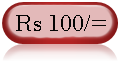Web Development : Starting from Concept, HTML, CSS, Web Site Develpment to Website Launching.
Written by: Sanjay Srivastava
B.Tech (Honours);IIT Kharagpur
PMP ; PMI USA

Class - VIII - Maths:- INDEX

1. Squares & Square Roots
2. Cubes & Cube Roots
4. Profit Loss & Discount
5. Compound Interest
6. Algebraic Identities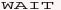7. Polynomials8. Linear Equations9. Parallel Lines10. Types of Quadrilaterals11. Construction of Quadrilaterals12. Circles13. Areas14. Surface Areas15. Volumes16. StatisticsClass - VIII - Maths

## What is Compound Interest Formula (Page 1/3)

Important Tips (1) Simple Interest = (P * T * R)/100
[ P=Principal; R=Rate of interest; T=Time]
Please make sure that the both 'Time' and 'Rate of interest' are in the same reference .i.e. years / months / days
C.I. = Compound Interest;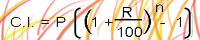n=No of period

Question - Type 1 -
Find the compound interest on Rs 10000/- for 3 years @ 10 % annually, by simple interest and compound interest formula.

Refer the formulas shown in left side.
By Simple Interest Method - Since the question is for compound interest annually, therefore we have to calculate annually.
(a) Interest for 1st year = 10000 * 1 * 10/100 = 1000
P after 1 year = 10000+1000=11000
(a) Interest for 2nd year = 11000 * 1 * 10/100 = 1100
P after 1 year = 11000+1100=12100
(a) Interest for 3rd year = 12100 * 1 * 10/100 = 1210
P after 1 year = 12100+1210=13310
Therefore Interest = 13310 - 10000 = Rs 3310

By Compound Interest Method - P=10000 R=10 n=3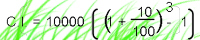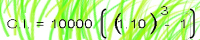= 10000 [(1.331)-1] = 10000 * .331 = Rs 3310